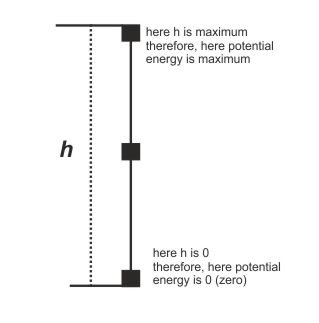Potential Energy - Fundamentals - Physics
12-10-2018    551 times### potential energy

Def. The energy possessed by a body by virtue of its specific position (or changed configuration) is called the potential energy.

### Measurement of energy

Let a body with the mass m lifted from the ground (earth surface) to a vertical height h against the gravitational energy. The total work done by lifting this body is store into the body as potential energy. Now, if we release the body then it fall down due to the potential energy store into the body.

Therefore,The potential energy of the body at the height h = work done to lifting the body at height h

Now we know,

work = Force X displacement
Force = weight of the body = m.g    and
displacement = height of the body from earth surface = h

That is, work= m.g.h

The potential energy of the body on earth surface is 0(zero) and potential energy of a body under the earth (mine) is negative.

When a body thrown vertically upwards, It is rise up, so that the height of the body from the ground increases, hence potential energy is increases. On the other hand when a body is dropped from the height its height is decrease from the earth surface, therefore potential energy is decrease of that body. And it is become zero on earth surface.